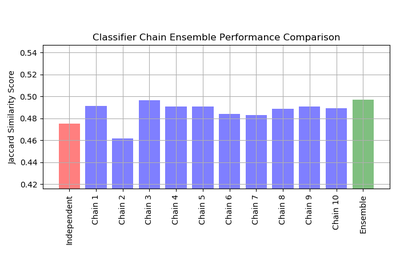# `sklearn.multioutput`.ClassifierChain¶

class `sklearn.multioutput.``ClassifierChain`(base_estimator, order=None, cv=None, random_state=None)[source]

A multi-label model that arranges binary classifiers into a chain.

Each model makes a prediction in the order specified by the chain using all of the available features provided to the model plus the predictions of models that are earlier in the chain.

Parameters: base_estimator : estimator The base estimator from which the classifier chain is built. order : array-like, shape=[n_outputs] or ‘random’, optional By default the order will be determined by the order of columns in the label matrix Y.: ```order = [0, 1, 2, ..., Y.shape - 1] ``` The order of the chain can be explicitly set by providing a list of integers. For example, for a chain of length 5.: ```order = [1, 3, 2, 4, 0] ``` means that the first model in the chain will make predictions for column 1 in the Y matrix, the second model will make predictions for column 3, etc. If order is ‘random’ a random ordering will be used. cv : int, cross-validation generator or an iterable, optional ( default=None) : Determines whether to use cross validated predictions or true labels for the results of previous estimators in the chain. If cv is None the true labels are used when fitting. Otherwise possible inputs for cv are: integer, to specify the number of folds in a (Stratified)KFold, An object to be used as a cross-validation generator. An iterable yielding train, test splits. random_state : int, RandomState instance or None, optional (default=None) If int, random_state is the seed used by the random number generator; If RandomState instance, random_state is the random number generator; If None, the random number generator is the RandomState instance used by np.random. The random number generator is used to generate random chain orders. classes_ : list A list of arrays of length `len(estimators_)` containing the class labels for each estimator in the chain. estimators_ : list A list of clones of base_estimator. order_ : list The order of labels in the classifier chain.

References

Jesse Read, Bernhard Pfahringer, Geoff Holmes, Eibe Frank, “Classifier Chains for Multi-label Classification”, 2009.

Methods

 `decision_function`(X) Evaluate the decision_function of the models in the chain. `fit`(X, Y) Fit the model to data matrix X and targets Y. `get_params`([deep]) Get parameters for this estimator. `predict`(X) Predict on the data matrix X using the ClassifierChain model. `predict_proba`(X) Predict probability estimates. `score`(X, y[, sample_weight]) Returns the mean accuracy on the given test data and labels. `set_params`(**params) Set the parameters of this estimator.
`__init__`(base_estimator, order=None, cv=None, random_state=None)[source]
`decision_function`(X)[source]

Evaluate the decision_function of the models in the chain.

Parameters: X : array-like, shape (n_samples, n_features) Y_decision : array-like, shape (n_samples, n_classes ) Returns the decision function of the sample for each model in the chain.
`fit`(X, Y)[source]

Fit the model to data matrix X and targets Y.

Parameters: X : {array-like, sparse matrix}, shape (n_samples, n_features) The input data. Y : array-like, shape (n_samples, n_classes) The target values. self : object Returns self.
`get_params`(deep=True)[source]

Get parameters for this estimator.

Parameters: deep : boolean, optional If True, will return the parameters for this estimator and contained subobjects that are estimators. params : mapping of string to any Parameter names mapped to their values.
`predict`(X)[source]

Predict on the data matrix X using the ClassifierChain model.

Parameters: X : {array-like, sparse matrix}, shape (n_samples, n_features) The input data. Y_pred : array-like, shape (n_samples, n_classes) The predicted values.
`predict_proba`(X)[source]

Predict probability estimates.

Parameters: X : {array-like, sparse matrix}, shape (n_samples, n_features) Y_prob : array-like, shape (n_samples, n_classes)
`score`(X, y, sample_weight=None)[source]

Returns the mean accuracy on the given test data and labels.

In multi-label classification, this is the subset accuracy which is a harsh metric since you require for each sample that each label set be correctly predicted.

Parameters: X : array-like, shape = (n_samples, n_features) Test samples. y : array-like, shape = (n_samples) or (n_samples, n_outputs) True labels for X. sample_weight : array-like, shape = [n_samples], optional Sample weights. score : float Mean accuracy of self.predict(X) wrt. y.
`set_params`(**params)[source]

Set the parameters of this estimator.

The method works on simple estimators as well as on nested objects (such as pipelines). The latter have parameters of the form `<component>__<parameter>` so that it’s possible to update each component of a nested object.

Returns: self :

## Examples using `sklearn.multioutput.ClassifierChain`¶Classifier Chain